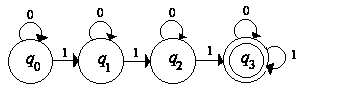GFG App
Open AppBrowser
Continue

# How To Write Transition Sequence of an Automata?

Automata is generally a machine that accepts the strings of a language L over an input alphabet Σ. it is the kind of machine which takes some string as input and this input goes through a finite number of states and may enter in the final state. The State is represented by circles, the Transitions is represented by arrows and the final state is represented by double circle.

There are majorly three methods to define an automata i.e. Transition graph, Transition table and Transition sequence.

In transition graph, we generally define the automata in the form a graph or a diagram in which all the transitions from states to states are mentioned. whereas in Transition table we made a table in which one side we take input symbols and other side will be of states. initial state is mentioned by an arrow towards it and final states are mentioned with a circle in the transition table, refer this article to know more about “Transition table in Automata“.

Now the third way to represent an Automata is Transition sequence. The transition sequence can be represented as ∫(current state, current input symbol) -> next state. For instance if q0 is the current state and 0 is the current input symbol, then the transition function is ∫(q0, 0) -> q1, here at q0 state it takes input 0 and goes to q1 state.

Let’s write a transition sequence of a given Automata:Since q0 is our initial state so we start from there, first we write the transition sequence for q0 with both of its input symbols, it will be like ∫(q0, 0) -> q0 it means that, at q0 it takes input 0 and goes to same q0 state. similarly at q0, it takes input 1 and goes to q1 state, then its transition sequence will be written like ∫(q0, 1) -> q1.

In a similar fashion, we do this for q1 state, at q1 state it take input of 0 and goes to same q1 state and when it accepts 1 as input symbol at q1 state then it goes to q2 state. so the transition sequence will be like ∫(q1, 0) -> q1 and ∫(q1, 1) -> q2.

now at q2 state, when it take input symbol 0 it goes back to q2 again and when it accepts 1 as input symbol then goes to q3. the transition sequence will be ∫(q2, 0) -> q2 and ∫(q3, 1) -> q3.

same process we follow for the final state(q3) as well. at q3 state, when it take input symbol 0 it goes back to q3 again and when it accepts 1 as input symbol again it goes to q3. the transition sequence will be ∫(q3, 0) -> q3 and ∫(q3, 1) -> q3.

All the transitions sequence of above Automata are as follows:

```∫(q0, 0) -> q0     ∫(q0, 1) -> q1
∫(q1, 0) -> q1     ∫(q1, 1) -> q2
∫(q2, 0) -> q2     ∫(q3, 1) -> q3
∫(q3, 0) -> q3     ∫(q3, 1) -> q3```

That’s it, this is how we write a transition sequence of an Automata.

My Personal Notes arrow_drop_up